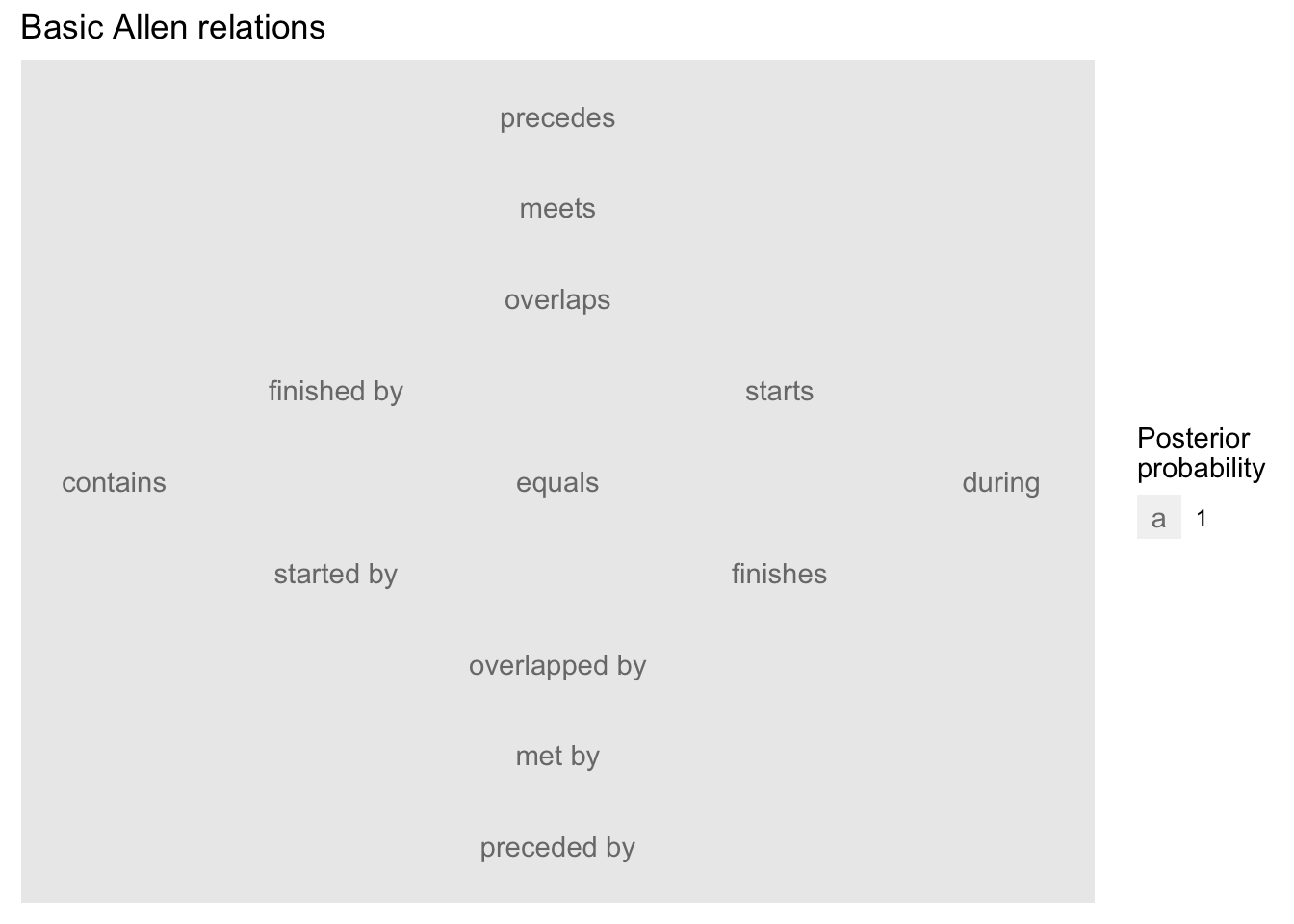1. ArchaeoPhases : Allen’s Interval Algebra

`=============`

```{r, echo = FALSE, message = FALSE} knitr::opts_chunk\$set(comment = ““) options(width = 120, max.print = 20) library(ArchaeoPhases)

```

## Introduction

Version `ArchaeoPhases 1.6` adds facilities to compute Allen’s interval algebra. This vignette provides an overview of the algebra and illustrates how it might be used analytically and empirically, given MCMC output from a Bayesian calibration.

## Overview of Allen’s Interval Algebra

Allen’s interval algebra identifies 13 basic relations that are distinct, exhaustive, and qualitative. The algebra is defined for definite intervals whose endpoints are single values. It can also be used with indefinite intervals whose endpoints are not single values.

It is conventional to express an Allen relation with a notation that indicates two intervals and a set of relations. For example, given definite intervals A and B, where A precedes B, their Allen relation can be represented as A/(p)/B. Given indefinite intervals C and D, where C starts before D starts and ends before D ends, an Allen relation that expresses this incomplete information is C/(pmo)/D. Note that the Allen set (pmo) in the relation of C and D is a superset of the Allen set (p) in the relation of A and B. In this circumstance, when two Allen sets are related as superset/subset, the relation indicated by the superset is weaker and the relation indicated by the subset is stronger. An Allen relation thus provides a precise vocabulary for characterizing the state of knowledge about the relation between two intervals, regardless of whether the intervals are definite or indefinite.

 Relation Converse precedes (p) (P) preceded by meets (m) (M) met by overlaps (o) (O) overlapped by finished by (F) (f) finishes contains (D) (d) during starts (s) (S) started by equals (e)

It is often useful to visualize the basic Allen relations as a Nökel lattice. In a Nökel lattice, the immediate neighbors of a relation differ from it in the placement of a single interval end-point.

``````## Load ArchaeoPhases
library(ArchaeoPhases)``````
``## Loading required package: coda``
``## Loading required package: hdrcde``
``## This is hdrcde 3.4``
``````## Plot the basic Allen relations
allen_illustrate("basic") ````````````##    x y result          node                 title
## p  0 8      1      precedes Basic Allen relations
## m  0 7      1         meets Basic Allen relations
## o  0 6      1      overlaps Basic Allen relations
## F -1 5      1   finished by Basic Allen relations
## s  1 5      1        starts Basic Allen relations
## D -2 4      1      contains Basic Allen relations
## e  0 4      1        equals Basic Allen relations
## d  2 4      1        during Basic Allen relations
## S -1 3      1    started by Basic Allen relations
## f  1 3      1      finishes Basic Allen relations
## O  0 2      1 overlapped by Basic Allen relations
## M  0 1      1        met by Basic Allen relations
## P  0 0      1   preceded by Basic Allen relations``````

## Analytic Inquiry with the Algebra

Interval algebra is carried out with a composition function that returns the relation of two intervals given their relations to a common third interval. As implemented here, the function expects the relation of one interval to the common interval and the relation of the common interval to the remaining interval. The function also accepts a title for the resulting Nokel lattice.

In this example, the composition function deduces that the interval represented by a context inferior to a reference context precedes the interval represented by a context superior to the reference context.

``````## Load ArchaeoPhases
library(ArchaeoPhases)
## Plot lattice for two contexts on the same line of a Harris matrix
allen_analyze("m", "m", "Composite relation of two contexts on the same line") ``````